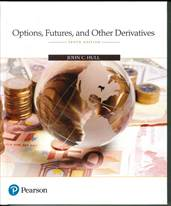Options, Futures, and
Other Derivatives,
Tenth Edition

Errata in Book:

Page 62: lines 8 and 9. Replace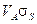by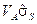and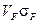by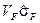. (This was kindly pointed out by Sebastien Bossu.)

Page 62: line 14. “Example 3.5” should be “Example 3.3” (This was kindly pointed out by Hendrik Hofmann)

Page 89: line 1: “Table 4.3” should be “Table 4.5  (This was kindly pointed out by Dan Pecur)

Page 91: In Business Snapshot 4.1 and on first line of last paragraph, replace “Table 4.5” with “Table 4.6” (This was kindly pointed out by Emilio Barone.)

Page 139: First lines of Quotes section: Replace “Treasury note” with “Ultra T-bond” Also replace 123.0390625 by 121.0390625 on last line of this subsection (This was kindly pointed out by Emilio Barone.)

Page 144: line 10. Replace “Thus, the settlement price of 99.725 for the June 2013 contract” with “Thus, the May 3 trade price of 99.330 for the June 2016 contract”(This was kindly pointed out by Raúl Gimeno.)

Page 167: Example 7.2, line 9: replace “LIBOR is paid and 5% is received” with “LIBOR is received and 5% is paid”. (This was kindly pointed out by Emilio Barone.)

Page 171: last two lines: replace USD 156,000 (=1.3% of 12 million) with USD 195,000 (=1.3% of \$15 million). (This was kindly pointed out by Emilio Barone.)

Page 340: line 5. Replace “Section 26.15” with “Section 26.16”. (This was kindly pointed out by Emilio Barone.)

Page 349: Problem 15.33: second equation: Replace “KN(-d1)-E(V)N(-d2)” with Replace “KN(-d2)-E(V)N(-d1)” (This was kindly pointed out by Emilio Barone.)

Page 383: Example 18.3: line 8: Replace 3.22% by 2.22%. (This was kindly pointed out by Emilio Barone.)

Page 515: Change equation in the middle of the page from DP = −0.05f1−3.88f2 to DP = 0.05f1−3.88f2 (i.e. delete minus sign).

Page 549: line before last equation: replace 0.01441 by 0.01345. (This was kindly pointed out by Emilio Barone.)

Page 560: In first paragraph Problem 24.29 should be Problem 24.31 and Problem 24.30 should be Problem 24.32. (This was kindly pointed out by Emilio Barone.)

Page 565: line 11: 2.4593 should be 2.4573 in two places. ((This was kindly pointed out by Hendrik Hofmann)

Page 608: Line before equation (26.3): Change “by equations (18.9) and (18.10)” to “by equations (18.7) and (18.8)”

Page 630: First line after equations: Replace F = K by F0 = K. Also problem reference at the end of the next paragraph should be Problem 27.23 not Problem 27.24. (This was kindly pointed out by Emilio Barone.)

Page 631: Five lines form end: “all or nothing” should be “cash or nothing” (This was kindly pointed out by Emilio Barone.)

Page 648: The journal for the Milanov et al article is Journal of Fixed Income (This was kindly pointed out by Hendrik Hofmann)

Page 649: First equation. Replace VT by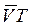. (This was kindly pointed out by Emilio Barone.)

Page 661: Two lines after equation (28.24): Replace “current swap rate” by “current forward swap rate” (This was kindly pointed out by Emilio Barone.)

Page 680: line after third equation: Reference to Chapter 27 should be to Chapter 28. (This was kindly pointed out by Emilio Barone.)

Page 684: Subsection Negative Rate, third line: Replace RK by sK in two places. (This was kindly pointed out by Emilio Barone.)

Page 688: Problem 29.22: Delete “with LIBOR discounting” on second line (This was kindly pointed out by Emilio Barone.)

Page 692: Example 30.1, line 6: Replace 1/1.043 by 1/1.0410. Also in last paragraph and last equation 9.8710 should be 9.8910. (This was kindly pointed out by Emilio Barone.)

Page 708: Last paragraph before Bond Price Process subsection: reference to Table 4.6 should be to Table 4.7. (This was kindly pointed out by Emilio Barone.)

Page 710: footnote 5: reference to Problem 31.16 should be to Problem 31.17. (This was kindly pointed out by Emilio Barone.)

Page 734: Delete references to Tables 29.1 and 29.2 in last paragraph of Section 32.6 and in footnote 16. Also reference to Section 29.5 in first line of Section 32.7 should be to Section Section 29.4. (This was kindly pointed out by Emilio Barone.)

Page 842: In the Application Builder subsection replace DG 300 by DG400 in three places (lines 1-3) and Section 26.16 by 26.17 in one place (3 lines from end) (This was kindly pointed out by Emilio Barone.)

Errata in Solutions Manual

Page 23: Problem 4.3. In first equation, last term on the left hand side should be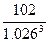and in the second equation the second term on the left hand side should be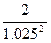.

Page 35: Problem 5.13. The June settlement price is 0.94015, not 0.94105. As a result 0.23% should be changed to 0.32% in two places and 0.92% should be changed to 1.29%. (This was kindly pointed out by Emilio Barone.)

Page 44: line 5: 5e-0.1370×0.1165 should be 6.5e-0.01370×0.1165 (This was kindly pointed out by Nicolas Mougeot.)

Page 199, Problem 25.2, line 3 of answer. 0.060 should be 0.0060.

Page 247: Problem 31.4. In the expression for A(0,5),should be. (This was kindly pointed out by Emilio Barone.)

Page 254: Problem 32.8. First line after first equation. Replace (31.16) and (31.17) with (32.7) and (32.8). (This was kindly pointed out by Emilio Barone.)

Page 257: Problem 32.15. First line. Replace equation (32.15) with equation (32.6). (This was kindly pointed out by Emilio Barone.)